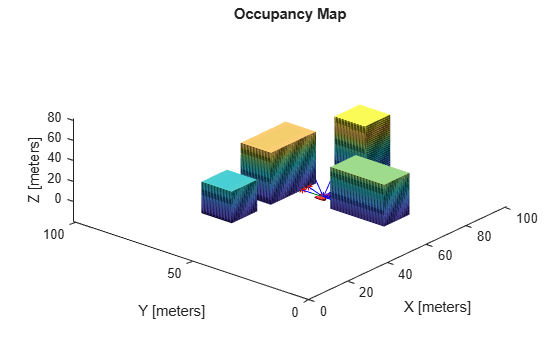# rayIntersection

Find intersection points of rays and occupied map cells

## Syntax

``[intersectionPts,isOccupied] = rayIntersection(map3D,sensorPose,directions,maxrange)``
``[intersectionPts,isOccupied] = rayIntersection(map3D,sensorPose,directions,maxrange,ignoreUnknown)``

## Description

example

````[intersectionPts,isOccupied] = rayIntersection(map3D,sensorPose,directions,maxrange)` returns intersection points of rays in the specified map, `map3D`. Rays emanate from the specified `sensorPose` at the given orientations, `directions`. Intersection points are returned in the world coordinate frame. Use `isOccupied` to determine if the intersection point is at the sensor max range or if it intersects an obstacle.```
````[intersectionPts,isOccupied] = rayIntersection(map3D,sensorPose,directions,maxrange,ignoreUnknown)`additionally accepts optional arguments for the sensors max range and whether to ignore unknown values. By default, the rays extend to the map boundary and unknown values are ignored.```

## Examples

collapse all

Import a 3-D occupancy map.

`map3D = importOccupancyMap3D("citymap.ot")`
```map3D = occupancyMap3D with properties: ProbabilitySaturation: [1.0000e-03 0.9990] Resolution: 1 OccupiedThreshold: 0.6500 FreeThreshold: 0.2000 ```

Inflate the occupied areas by a radius of 1 m. Display the map.

```inflate(map3D,1) show(map3D)```

Find the intersection points of rays and occupied map cells.

```numRays = 10; angles = linspace(-pi/2,pi/2,numRays); directions = [cos(angles); sin(angles); zeros(1,numRays)]'; sensorPose = [55 40 1 1 0 0 0]; maxrange = 15; [intersectionPts,isOccupied] = rayIntersection(map3D,sensorPose,directions,maxrange)```
```intersectionPts = 10×3 55.0000 32.0000 1.0000 57.9118 32.0000 1.0000 61.7128 32.0000 1.0000 67.9904 32.5000 1.0000 69.0000 37.5314 1.0000 69.0000 42.4686 1.0000 67.9904 47.5000 1.0000 64.6418 51.4907 1.0000 58.2757 49.0000 1.0000 55.0000 49.0000 1.0000 ```
```isOccupied = 10×1 1 1 1 -1 1 1 -1 -1 1 1 ```

Plot the intersection points and rays from the pose.

```hold on plotTransforms(sensorPose(1:3),sensorPose(4:end),... 'FrameSize',5,'MeshFilePath','groundvehicle.stl') % Vehicle sensor pose for i = 1:numRays plot3([sensorPose(1),intersectionPts(i,1)],... [sensorPose(2),intersectionPts(i,2)],... [sensorPose(3),intersectionPts(i,3)],'-b') % Plot rays if isOccupied(i) == 1 plot3(intersectionPts(i,1),intersectionPts(i,2),intersectionPts(i,3),'*r') % Intersection points end end```## Input Arguments

collapse all

3-D occupancy map, specified as a `occupancyMap3D` object.

Position and orientation of sensor, specified as an ```[x y z qw qx qy qz]``` vector. The vehicle pose is an xyz-position vector with a quaternion orientation vector specified as ```[qw qx qy qz]```.

Orientation of rays emanating from the sensor relative to the sensor coordinate frame, specified as an n-by-3 `[dx dy dz]` matrix or n-by-2 `[az el]` matrix.

• `[dx dy dz]` is a directional vector in xyz-coordinates.

• `[az el]` is a vector with azimuth angle, `az`, measured from the positive x direction to the positive y direction, and elevation angle from the xy-plane to the positive z-direction in sensor coordinate frame.

Maximum range of laser range sensor, specified as a scalar in meters. Range values greater than or equal to `maxrange` are considered free along the whole length of the ray, up to `maxrange`.

Interpret unknown values in the map as free or occupied specified as `1` or `0`. Set this value to `0` to assume unknown values are occupied.

## Output Arguments

collapse all

Intersection points, returned as n-by-3 matrix of ```[x y z]``` points in the world frame, where n is the length of `directions`.

Occupancy status of ray end points, returned as a vector of zeroes and ones. Use `isOccupied` to determine if the intersection point is at the sensor max range or if it intersects an obstacle.

## Version History

Introduced in R2020a